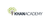# Class 11 Physics (India): Relative velocity in 2D

Product type
Total time

## Class 11 Physics (India): Relative velocity in 2DKhan Academy

Need more information? Get more details on the site of the provider.### Description

How fast is the sheep moving as seen by the lion? In previous videos, we have already seen how to calculate relative velocity in 1D. In this video let's extend this concept to the next level, to 2D motion. Created by Mahesh Shenoy

## Class 11 Physics (India)

Let's learn, practice, and master topics of class 11 physics (NCERT) starting with kinematics and then moving to dynamics with Newton's laws of motion, work, energy, and power. Let's then use these as the foundation to learn about centre of mass, rotational motion, gravitation, solids, fluids, thermodynamics, and oscillations and waves.

## Relative motion in 2D

Let's learn how to solve problems involving relative velocity and frames of referen…

There are no frequently asked questions yet. If you have any more questions or need help, contact our customer service.

Didn't find what you were looking for? See also: Physics, Two Dimensional Design (2D Design), Teaching Skills, Biology, and Mathematics.

How fast is the sheep moving as seen by the lion? In previous videos, we have already seen how to calculate relative velocity in 1D. In this video let's extend this concept to the next level, to 2D motion. Created by Mahesh Shenoy

## Class 11 Physics (India)

Let's learn, practice, and master topics of class 11 physics (NCERT) starting with kinematics and then moving to dynamics with Newton's laws of motion, work, energy, and power. Let's then use these as the foundation to learn about centre of mass, rotational motion, gravitation, solids, fluids, thermodynamics, and oscillations and waves.

## Relative motion in 2D

Let's learn how to solve problems involving relative velocity and frames of reference when the motion happens on a plane.

## Topic: Science

Learn about all the sciences, from physics, chemistry and biology, to cosmology and astronomy, across hundreds of videos, articles and practice questions.

There are no reviews yet.